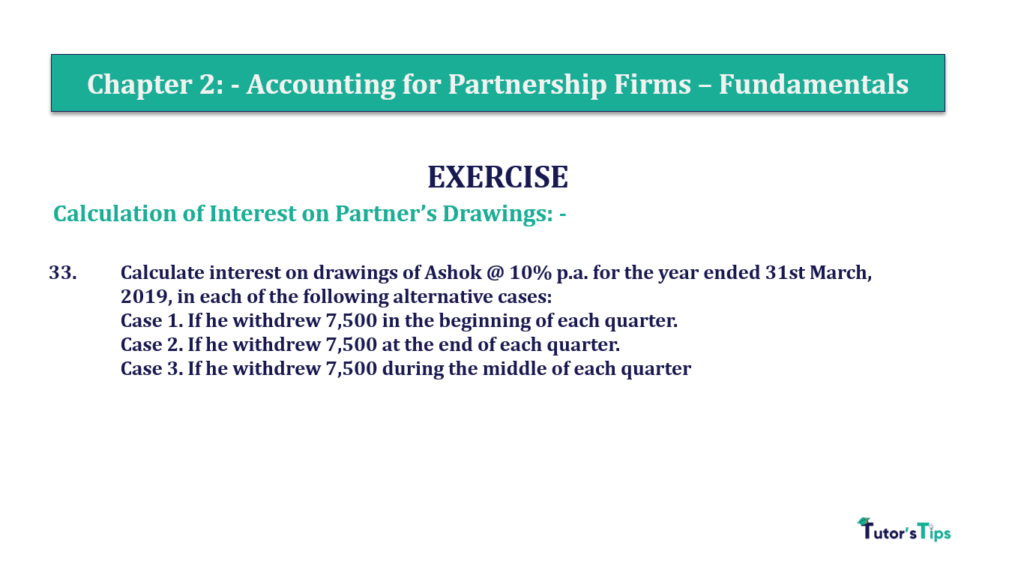# Question 33 Chapter 2 of +2-A – T.S. Grewal 12 Class Part – A Vol. 1Question 33 Chapter 2 of +2-A

Calculation of Interest on Partner’s Drawings: –

33. Calculate interest on drawings of Ashok @ 10% p.a. for the year ended 31st March, 2019, in each of the following alternative cases:
Case 1. If he withdrew 7,500 at the beginning of each quarter.
Case 2. If he withdrew 7,500 at the end of each quarter.
Case 3. If he withdrew 7,500 during the middle of each quarter

The solution of Question 33 Chapter 2 of +2-A

:

Case 1

Calculation of interest on partner’s Drawings
If partners withdrawal the same amount of drawing on a regular basis then we can calculate the interest on drawing with help of following formula: –
Interest on Drawing = Total Drawing X Rate of Interest X Period/12 Months
Rate of Interest = 10%
Period = the period/time will be calculated with the help of following formula: –

 Period = Time left after 1st withdrawal + Time left after Last withdrawal 2

Withdrawal at the beginning of each quarter = 7,500 p.m
It means 1st withdrawal has made on 01/04/2019
So, Time left from 01/04/2019 to 31/03/2020
are 12 Months
And last withdrawal has made on 01/01/2020
So, Time left from 01/01/2020 to 31/03/2020
are 3 Months
Now, put this time period in the formula

 Period = 12 + 3 2
 Period = 15 2
 Period = 7.5
 total Drawing = 7,500 * 4

=24,000 Multiplied by 6 because a number of withdrawal in 6 months will be 6.

 Interest on Ashok’s Drawing = 30,000 X 10 X 7.5 100 12

Interest on A’s Drawing = 1,875/-

Case 2

Calculation of interest on partner’s Drawings
Withdrawal at the end of each quarter = 7,500 p.m
It means 1st withdrawal has made on 30/06/2019
So, Time left from 30/06/2019 to 31/03/2020
are 9 Months
And last withdrawal has made on 31/03/2020
So, Time left from 31/03/2020 to 31/03/2020
are 0 Months
Now, put this time period in the formula

 Period = 9 + 0 2
 Period = 9 2
 Period = 4.5
 total Drawing = 7,500 * 4

=30,000

 Interest on Ashok’s Drawing = 30,000 X 10 X 4.5 100 12

Interest on A’s Drawing = 1,125/-

Case 3

Calculation of interest on partner’s Drawings
Withdrawal at the end of each quarter = 7,500 p.m
It means 1st withdrawal has made on 15/05/2019
So, Time left from 15/05/2019 to 31/03/2020
are 10.5 Months
And last withdrawal has made on 15/02/2020
So, Time left from 15/02/2020 to 31/03/2020
are 1.5 Months
Now, put this time period in the formula

 Period = 10.5 + 1.5 2
 Period = 12 2
 Period = 6
 total Drawing = 7,500 * 4

=30,000

 Interest on Ashok’s Drawing = 30,000 X 10 X 6 100 12

Interest on A’s Drawing = 1,500/-

Comment if you have any questions.

Also, Check out the solved question of previous Chapters: –

### T.S. Grewal’s Double Entry Book Keeping +2 (Vol. I: Accounting for Not-for-Profit Organizations and Partnership Firms)

• Chapter No. 1 – Financial Statement of Not-For-Profit Organisations
• Chapter No. 2 – Accounting for Partnership Firms – Fundamentals
• Chapter No. 3 – Goodwill: Nature and Valuation
• Chapter No. 4 – Change in Profit-Sharing Ratio Among the Existing Partners
• Chapter No. 5 – Admission of a Partner
• Chapter No. 6 – Retirement/Death of a Partner
• Chapter No. 7 – Dissolution of a Partnership Firm

### T.S. Grewal’s Double Entry Book Keeping (Vol. II: Accounting for Companies)

• Chapter No. 1 – Financial Statements of a Company
• Chapter No. 2 – Financial Statement Analysis
• Chapter No. 3 – Tools of Financial Statement Analysis – Comparative Statements and Common- Size Statements
• Chapter No. 4 – Accounting Ratios
• Chapter No. 5 – Cash Flow Statement